The potentiometer- Comparison of emf

# Objective

To compare the emf’s of two given primary cells using a potentiometer.

# Theory

## Potentiometer

Potentiometer is a device used to compare the e.m.f. (electromotive force) of two cells, to measure the internal resistance of a cell, and potential difference across a resistor. It consists of a long wire of uniform cross-sectional area and of 10 m in length. The material of wire should have a high resistivity and low temperature coefficient.  The wires are stretched parallel to each other on a wooden board. The wires are joined in series by using thick copper strips. A metre scale is also attached on the wooden board.

The potentiometer works on the principle that when a constant current flows through a wire of uniform cross sectional area, potential difference between its two points is directly proportional to the length of the wire between the two points.

## Electromotive force (e.m.f) of a cell

Electromotive force (emf) is a measurement of the energy that causes current to flow through a circuit.  It is the energy provided by a cell or battery per coulomb of charge passing through it. It can also be defined as the potential difference across the terminals of a cell, when no current flows through it. Electromotive force is also known as voltage, and it is measured in volts. Electromotive force is not truly a force; rather, it is a measurement of energy per unit charge.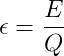, where E is the energy and Q is the charge.

Using a potentiometer, we can determine the emf of a cell by obtaining the balancing length l.  Here, the fall of potential along the length l of the potentiometer wire is equal to the emf of the cell, as no current is being drawn from the cell.

Then,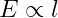or,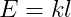;     where k is the potential gradient along the wire.

Thus it is possible to compare the emf’s of two given cells by measuring the respective balancing lengths l1 and l2.

ie;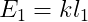and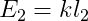or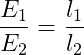# Learning Outcomes

• Students understand the potentiometer apparatus, its parts and how to use it.
• Students learn the concept of electromotive force in cells.
• Students are able to construct circuits based on circuit diagrams.
• Students understand the different components used in the experiment.